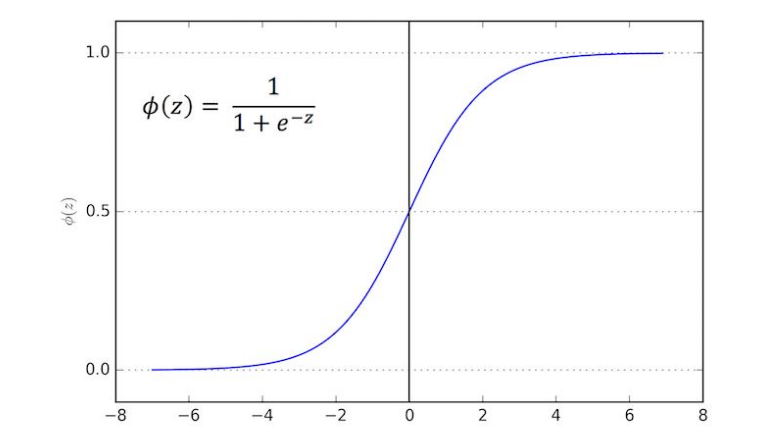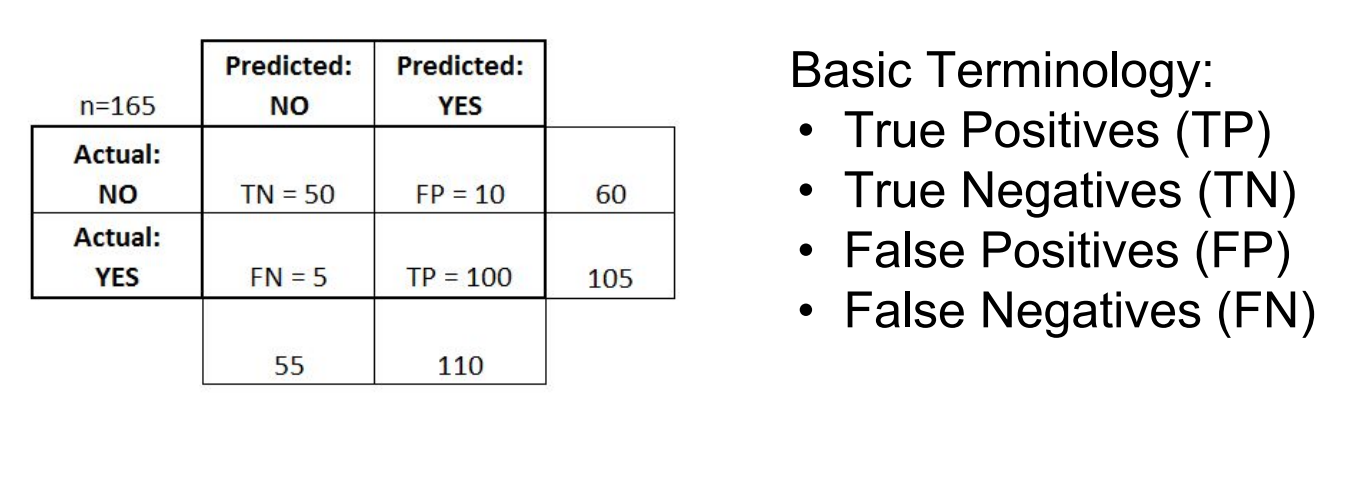# Logistic Regression

dougieduk·2022년 4월 1일
0

# Sigmoid Function

• takes in any value and outputs it to be between 0 and 1• in this case, we can set a cutoff point at 0.5
- p <0.5 → 0 / p>=0.5 → 1

# Evaluation

• confusion matrix can be used• Accuracy : (True positive+True Negative)/total
• Misclassification Rate : (False Positive + False Negative) / Total